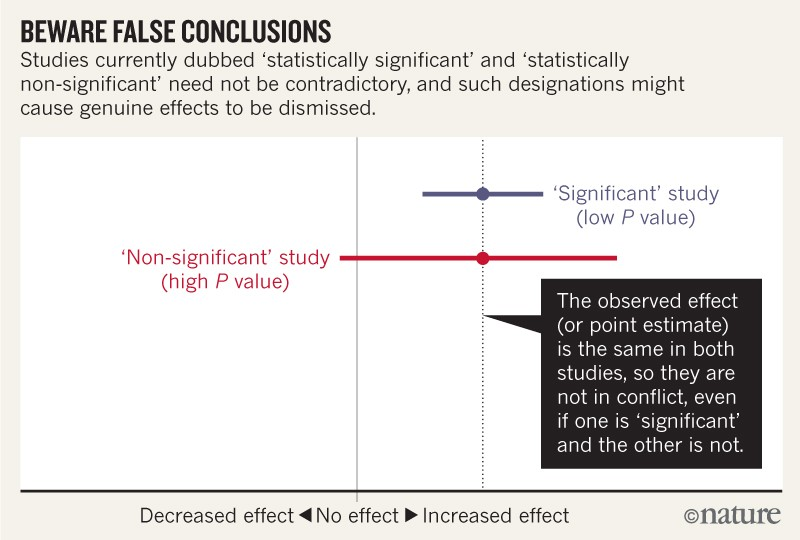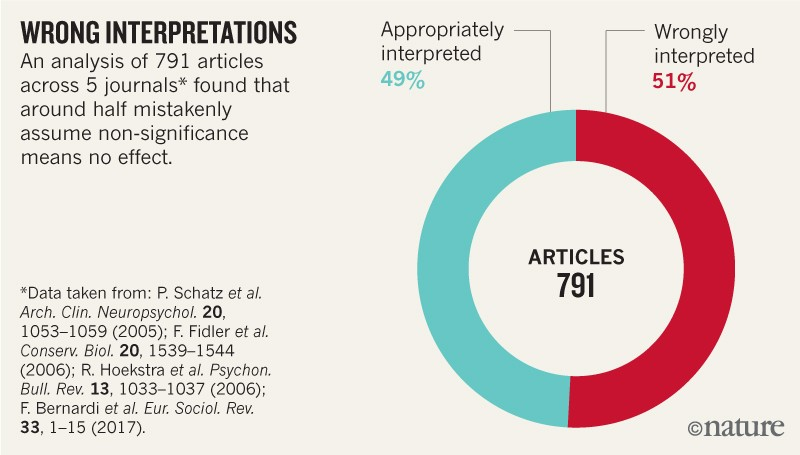# Statistical Significance in Epidemiology

## 2019/11/14

I was asked to give a mini-lesson in my international teaching assistant classes. With the help from Cyrus, my undergraduate student consultant, we put together a great tutorial on introducing statistical significance in epidemiology. During the preparation, I realized that these theories that we thought were quite basic in epidemiology are actually not easy to interpret. I post the note that Cyrus and I prepared. In this note, I would like to go over concepts related to statistical significance in the context of epidemiology. These concepts include significance levels, P-values, confidence intervals, and risk ratios. Before that, I will start with the null and alternative hypothesis, and then explain what a type one and type two error is.

Below is a data pipline, showing there are many stages to the design and analysis of a successful study 1:The definition of Null Hypothesis in Wiki is that

In inferential statistics, the null hypothesis is a general statement or default position that there is nothing new happening, like there is no association among groups, or no relationship between two measured phenomena.

In contrast with a Null Hypothesis, based on Wiki, an alternative hypothesis is

A position that states something is happening, a new theory is true instead of an old one.

To simplify this, I will use examples in cohort studies or clinical trials. In epidemiology, we sometimes use cohort studies and clinical trials to find our results. In a clinical trial, we first recruit a cohort of people, then divide them into two smaller groups, in which we assign an intervention (it could be medicine, device, program, et al.) to one of them, We follow them for a period of time to observe the proportions of people developed diseases among each group. In a cohort study, if we want to investigate, for example, the association between smoking and lung cancer, we recruit two groups of people, one with smokers and one group of nonsmokers. We follow over a period of time to track how many individuals in the study are diagnosed with lung cancer. In this, we do not intervene with development. In these study types, we test different hypotheses to compare the proportion of people who developed diseases among two groups, and to see if the difference is statistically significant. In the case of investigating the association between smoking and lung cancer, the null hypothesis is that smoking is not associated with lung cancer; and the alternative hypothesis is that smoking is associated with the risk of developing lung cancer.

In order to quantify how largely smoking will impact the risk of developing lung cancer, I will introduce a concept called risk ratio here. Our risk ratio is calculated by dividing the risk of developing lung cancer among smokers versus non-smokers. The null value, or the risk ratio according to our null hypothesis, is calculated to be one.

There are two types of errors that can occur among the hypothesis tests. There errors are known as type one and type two errors. A type one error is when we predict the alternative hypothesis to be correct when it is false. With a type two error, we accept the null hypothesis when it is false. A consequence of type one errors is a false positive. A consequence of type two errors is a false negative. The image below depicts an example of a pregnancy diagnosis to a man, versus a non-pregnant diagnosis with a women who is visibly pregnant.

When designing a study, we should set the probability of type one error that we can tolerate in the beginning. The majority of the epidemiologic study set the allowed type one error rate as 0.05. The rate is also called a significance level. Let’s go back to the smoking and lung cancer study, a significance level of 0.05 stands for a 5% risk of deciding that smoking is associated with lung cancer when there is not association.

Confidence intervals consist of a range of potential values of the unknown risk ratio of the population. The interval computed from one single study does not necessarily include the true value of the risk ratio.

One typical wrong interpretation is that a 95% confidence interval does not mean that for a given interval there is a 95% probability that the population parameter lies within the interval.

For example, if the true risk ratio for the association between smoking and lung cancer is 2. We conducted similar studies 100 times, 95 times our study would contain this true risk ratio.

P-value, invented by R.A. Fisher, stands for the probability of obtaining a result at least as extreme as the one that was actually observed in the epidemiology study, given that the null hypothesis is true. Let’s go back to our hypothetical smoking and lung cancer study. Suppose the hypothesis test generates a P-value of 0.04. I would interpret the p-value as follows: if smoking is not associated with lung cancer, 3% of studies will obtain the risk ratio at least as extreme as my observed risk ratio, due to random error or say chance.

However, we should never conclude that there is no association in a study just because the P-value is larger than 0.05, or that our confidence interval contains the null value. In this graph, the red bar predicts that there is no side effect of an anti-inflammatory drug, which in this case is atrial fibrillation. However, this result contradicted those of previous studies, which estimated a statistically significant outcome. In this study, the insignificant results had a risk ratio of 1.2 or a 20% increased risk of developing an irregular heartbeat among patients taking anti-inflammatory drugs, versus those unexposed. They also found a 95% confidence interval containing the null value, which would predict the study to be insignificant. Earlier researchers found the same risk ratio, but a much smaller confidence interval; meaning that their results were much more precise, and predicting that the anti-inflammatory drug is associated with increased risk of developing atrial fibrillation. When the confidence interval contains such as a serious increase of risk, it is absurd to say there is no association between the anti-inflammatory drug and atrial fibrillation. This graph shows how the precision of a study can mislead our interpretation of the results.Surveys of hundreds of articles have found that statistical non-significant results are interpreted as indicating “no difference” or “no association” in around half of studies.Sander Greenland et al. have summarized a list of common misinterpretations of p-values and confidence intervals 2.

In 2019, Valentin Amrhein, Sander Greenland, Blake McShane and more than 800 signatories call for an end to hyped claims and the dismissal of possibly crucial effects 3 . They advocate the abandon of categorizing p-value, since random error or chance alone can easily lead to large disparities in p-value, They gave an example in which two perfect replication studies have been conducted with 80% power for each of achieving p-value < 0.05; one study can obtain p-value < 0.01 whereas the other can have p-value > 0.30. They gave some guides on embracing uncertainty: (1) we need to know that any number within the confidence interval is reasonably compatible with the data, under the statistical assumptions that were used to compute the interval; (2) The values outside the interval are just less compatible compared to the values within the interval, and it is a wrong interpretation that confidence interval contains all possible values; (3) The point estimate is the most compatible with the data, and values near it are more compatible than those near the limits. For the confidence interval shown in the graph, we can interpret it as “Our results suggest a 20% increase in risk of new-onset atrial fibrillation in patients given the anti-inflammatory drugs. Nonetheless, a risk difference ranging from a 3% decrease, a small negative association, to a 48% increase, a substantial positive association, is also reasonably compatible with our data, given our assumptions.” (4) The 95% used to compute intervals is an arbitrary convention, imposing the dichotomization as a scientific standard, which perpetuates the problems of statistical significance; (5) Comparability hinge on the assumptions used to compute the interval, so it is a better practice to make assumptions as clear as possible and test the ones you can, and report all results.

Ronald L. Wasserstein et al. have given several Do’s and Don’t in the effort of moving to a world beyond “p < 0.05” 4 .

Don’t base your conclusions solely on whether an association or effect was found to be “statistically significant” (i.e., the p-value passed some arbitrary thresholds such as p < 0.05). Don’t believe that an association or effect exists just because it was statistically significant. Don’t believe that an association or effect is absent just because it was not statistically significant. Don’t believe that your p-value gives the probability that chance alone produced the observed association or effect or the probability that your test hypothesis is true. Don’t conclude anything about scientific or practical importance based on statistical significance (or lack thereof).

Their recommendation: Accept uncertainty. Be thoughtful, open, and modest. → ATOM

1. Leek JT, Peng RD. Statistics: P values are just the tip of the iceberg. Nature News. 2015 Apr 30;520(7549):612. Link
2. Greenland S, Senn SJ, Rothman KJ, Carlin JB, Poole C, Goodman SN, Altman DG. Statistical tests, P values, confidence intervals, and power: a guide to misinterpretations. European journal of epidemiology. 2016 Apr 1;31(4):337-50. Link
3. Amrhein V, Greenland S, McShane B. Scientists rise up against statistical significance. Link
4. Wasserstein RL, Schirm AL, Lazar NA. Moving to a world beyond “p< 0.05”. Link